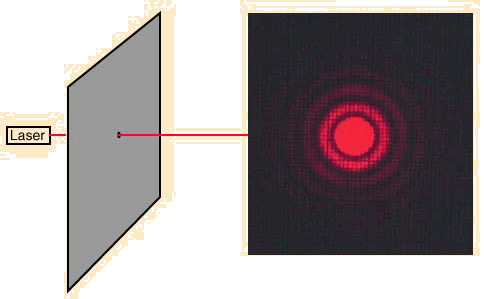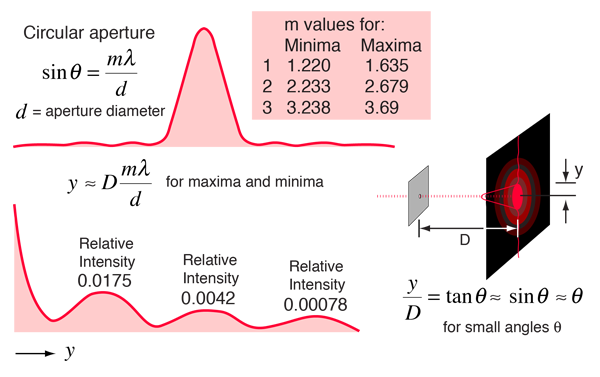# Circular Aperture DiffractionWhen light from a point source passes through a small circular aperture, it does not produce a bright dot as an image, but rather a diffuse circular disc known as Airy's disc surrounded by much fainter concentric circular rings. This example of diffraction is of great importance because the eye and many optical instruments have circular apertures. If this smearing of the image of the point source is larger that that produced by the aberrations of the system, the imaging process is said to be diffraction-limited, and that is the best that can be done with that size aperture. This limitation on the resolution of images is quantified in terms of the Rayleigh criterion so that the limiting resolution of a system can be calculated.

The aperture diffraction pattern above was photographed with Fuji Sensia 100ASA slide film and then digitized. With the time exposure necessary to show the side lobes, the central peak was washed out nearly white. The only retouching of the digital image was to paint in the washed out part of the central maximum (Airy's disc). The pinhole was made by placing aluminum foil on a glass plate, sticking a straight pin into the aluminum foil, and then rotating the foil. Several pinholes were made, and this one was the closest to being round.

 Mathematical description of aperture diffraction
 Example of corona around fog droplets
 Corona around Moon
Index

Diffraction concepts

Fraunhofer diffraction

 HyperPhysics***** Light and Vision R Nave
Go Back

# Circular Aperture DiffractionFor monochromatic light of wavelength λ = nm

incident upon a circular aperture of diameter d = micrometers,

d = x 10^ m,

and projected on a screen at distance D = m,

the displacement from the centerline on the screen is given by the relationship

Displacement y = Screen distance D*m value* wavelength / aperture diameter

 The active formula above can be used to calculate displacement y, wavelength, or aperture diameter by clicking on those quantities. You should enter the known data first, then click on the desired quantity. The entered data will not be forced to be consistent until you click on a quantity to calculate. Calculation of the displacement y will calculate the positions of all maxima and minima in the table below. Any quantity in that table can be changed for the purpose of calculating the other parameters. The active formula above is written as the small angle approximation, but the calculation makes use of the full trigonometric relationship.

This yields the following pattern of maxima and minima in the diffracted intensity.

 Order Minima Maxima m value Displacement y(cm) θ(degrees) m value Displacement y(cm) θ(degrees) 1 1.220 1.635 2 2.233 2.679 3 3.238 3.69
When light from a point source passes through a small circular aperture, it does not produce a bright dot as an image, but rather a diffuse circular disc known as Airy's disc surrounded by much fainter concentric circular rings. This example of diffraction is of great importance because the eye and many optical instruments have circular apertures. If this smearing of the image of the point source is larger that that produced by the aberrations of the system, the imaging process is said to be diffraction-limited, and that is the best that can be done with that size aperture. This limitation on the resolution of images is quantified in terms of the Rayleigh criterion so that the limiting resolution of a system can be calculated.
Index

Diffraction concepts

Fraunhofer diffraction

References
Jenkins and White
Sec 15.8

Hecht
Sec 10.2

 HyperPhysics***** Light and Vision R Nave
Go Back